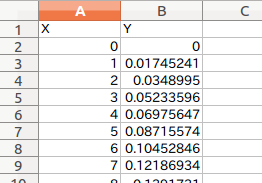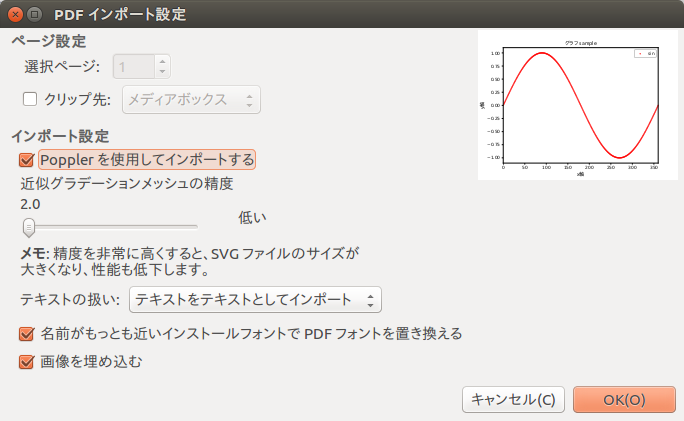# Graph Excel data with matplotlib

This is the basic code (for myself) to graph Excel data with matplot. I usually expand it as I like based on this.

environment ・ Ubuntu 16.04 ・ Python3.5.2 ・ Matplotlib 2.0.0 ・ Pandas0.19.2, numpy1.11.3 ・ Inkscape (used for eps → emf conversion)

## First, simplyA case where graph data (X-axis, Y-axis) is copied and used in the upper left (A1 cell) of the Excel sheet. It is assumed that the X-axis and Y-axis data you want to graph already exist. ・ I want to paste it in a report (Word), but I'm not happy with the Excel graph. ・ I'm dissatisfied with Excel's exponential notation Only in such a short time, I completely forget the plotting method.

I would like to describe the graphing with the data of any cell range of the existing Excel sheet in (2).

## Convert eps files to emf (extended metafile) using Inkscape

The output graph is saved as eps and png. The emf file is convenient when pasting it in a word document, so how to do it.

Inkscape is available from the command line, so use option -M

``````\$ inkscape sample.eps -M sample.emf
``````

I'd like to say, but it doesn't work with the following error ...

``````parser error : Start tag expected, '<' not found
^
** (inkscape:5467): WARNING **: Specified document sample.eps cannot be opened (does not exist or not a valid SVG file)
``````

I'll put this area off I have to make an emf file and put it on the report. It is a method to open inkscape and convert it normally.

(Added 2017.2.21) ↓ I wrote the solution (using Inkscape) here Convert matplotlib graph to emf file format

-Open the eps file in Inkscape and select emf in "Save As".

If you are using Japanese for the graph Characters are garbled if Import using Poppler in [Import Settings] is not checked. (I don't know what Poppler is ...)I wish I could save emf directly, but I'm not sure.

Postscript (2017.2.21) According to Piyopiyo no Hibi, the output function of emf has been deleted. What ...

``````matplotlib-1.3.In 1 document"change point"There was an explanation.
It was written like this.
"By organizing the features, the emf output feature has been removed from this version."
What's that...
``````

The current version of matplotlib is 2.0.0, so just in case I checked it by the method below.

``````import matplotlib.pyplot as plt
fig = plt.figure()
print(fig.canvas.get_supported_filetypes())
``````

If you look it up in

``````'pdf': 'Portable Document Format',
'ps': 'Postscript',
'rgba': 'Raw RGBA bitmap',
'pgf': 'PGF code for LaTeX',
'svgz': 'Scalable Vector Graphics',
'svg': 'Scalable Vector Graphics',
'raw': 'Raw RGBA bitmap',
'eps': 'Encapsulated Postscript',
'png': 'Portable Network Graphics'
``````

This seems to be the outputable file type in the current environment.

## code

So here is the code: From DataFrame of Excel file (xls, xlsx) read by pandas All you have to do is extract the x-axis and y-axis data and throw it into the plot.

There are two ways to extract, such as specifying by column or by label name.

#### `graph.py`

``````
# coding: utf-8

import numpy as np
import pandas as pd
import matplotlib.pyplot as plt
from matplotlib.font_manager import FontProperties

#Specify the location of the font file ubuntu(C:\Windows\Fonts\Any Font)
fp = FontProperties(fname="/usr/share/fonts/truetype/fonts-japanese-gothic.ttf")

####################################################################
filepath='~/Desktop/sample.xls' #Xls file location

#Read x-axis and Y-axis data from the data frame

#Specified by column
x=df[]
y=df[]

#When specifying by label
#x=df['X']
#y=df['Y']

####################################################################
#Graph
####################################################################
fig = plt.figure()

ax1.set_yscale('linear')
#ax1.set_yscale('log')

ax1.set_ylim(-1.1, 1.1)
ax1.set_xlim(0,360)

ax1.set_title("Graph sample",fontdict = {"fontproperties": fp},fontsize=12)
ax1.set_xlabel("x axis",fontdict = {"fontproperties": fp},fontsize=12)
ax1.set_ylabel("y axis",fontdict = {"fontproperties": fp},fontsize=12)

#ax1.plot(x, y,'red',label='sin')
ax1.scatter(x, y,s=1,c='r',label='sin')
ax1.legend(loc="upper right")

#Save file Save as both png and eps
plt.savefig("sample.png ")
plt.savefig("sample.eps")
plt.show()
``````